Assignment 2: Sphere Tracing Distance Estimators

# Description

PBRT has a small number of simple intersectable shapes, namely triangles and various simple quadrics. More complicated shapes must be approximated by building them out of these simple primitives.

In this assignment, we will extend PBRT to support a direct intersection method for arbitrarily complicated shapes.

The method we will be using is called sphere-tracing (often referred to as "ray-marching") of distance estimators, and has gained significant popularity among the "demo-scene" and technical artists for its ability to intersect extraordinarily intricate geometric structure with very low memory requirements.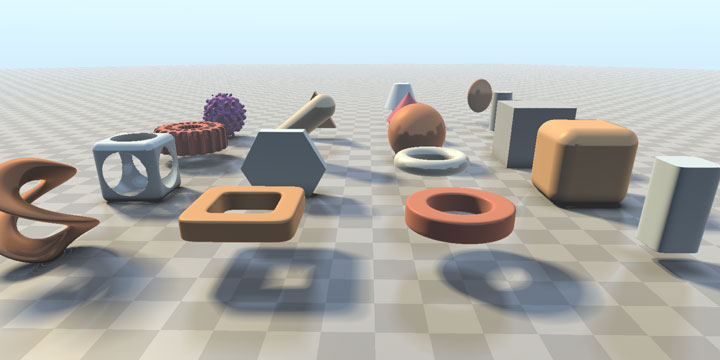Above is an example of various distance estimators ("primitives" in common parlance, "Shapes" in pbrt's terminology), click through the link if your computer has a decent GPU to see the scene sphere-traced in realtime.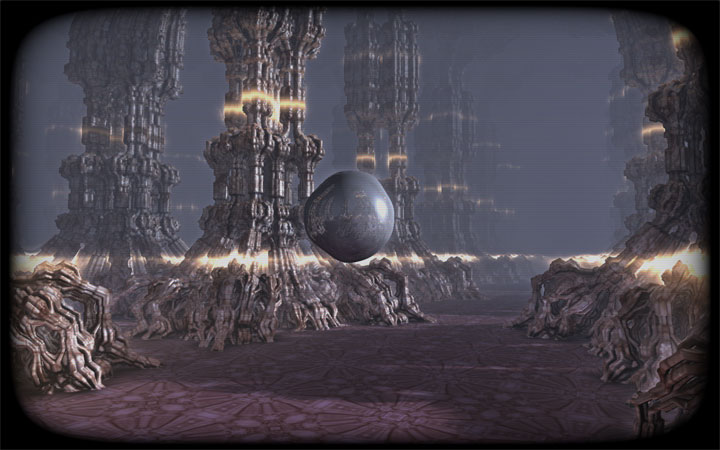Above is an example of a more complicated scene (also rendered in realtime) using transformations of various distance estimators.

In the links above, and in most popular sphere-tracing implementations, the sphere-tracing implementation is specialized to the scene, and uses non-physically-based hacks to approximate various lighting effects to speed up the computation so that it can run in realtime. We will be taking a more principled approach, creating a generic DistanceEstimator Shape that integrates well with the rest of pbrt, so that we can use distance estimators in our physically-based framework.

# Step 0: GitHub Setup

You will be using the GitHub repository you created for assignment 1 again for this assignment (and future assignments).

Create a new branch based on your unmodified master branch:

 git checkout -b assignment2 master


Be sure to make your changes in this branch as you work through the assignment.

There is a lot of formalism and an entire section on antialiasing that is not useful for the purpose of this project. The meat of the algorithm is actually entirely contained in the (very short) Figure 1. For a more modern explanation of the algorithm and of distance estimators, see Step 3.

# Step 2: Extend PBRT

First download the PBRT scene files from here and extract them into scenes/assignment2.

This is the first time you will be extending the actual pbrt code, so we will start with a small change. You will be extending pbrt with a new Shape subclass, called DistanceEstimator, which you should declare and implement in new files: shapes/distanceestimator.h and shapes/distanceestimator.cpp.

class Shape {
public:
// Shape Interface
Shape(const Transform *ObjectToWorld, const Transform *WorldToObject,
bool reverseOrientation);

//You will need to implement the 4 virtual functions below
virtual Bounds3f ObjectBound() const = 0;
virtual bool Intersect(const Ray& ray, Float *tHit,
SurfaceInteraction *isect,
bool testAlphaTexture = true) const = 0;
virtual Float Area() const = 0;
virtual Interaction Sample(const Point2f &u, Float *pdf) const = 0;

// We can use the default implementation
// of all the functions below this point
virtual ~Shape();
virtual bool IntersectP(const Ray& ray, bool testAlphaTexture = true) const;
virtual Bounds3f WorldBound() const;
virtual Float Pdf(const Interaction &) const;
virtual Interaction Sample(const Interaction& ref, const Point2f &u,
Float *pdf) const;
virtual Float Pdf(const Interaction& ref, const Vector3f& wi) const;
virtual Float SolidAngle(const Point3f& p, int nSamples = 512) const;
};


You only need to implement the four pure virtual functions from Shape specified above, the rest have acceptable default implementations. We will also only stub out the Sample function: it is only needed for using the shape as an area light, which we will not do in this project.

A quick note: IntersectP() is used by pbrt when casting shadow rays; since shadow rays only need to know if there was any hit before their tMax value, pbrt does not need the tHit value or a SurfaceInteraction describing the hitpoint. The default implementation of IntersectP() just calls Intersect() with nullptr for tHit and isect; this should generically work, although it may lead to doing extra work that is thrown away. However, it is undefined behavior to try and write to the address of a nullptr, so be sure not to write into tHit or isect if they are null or you may find yourself debugging strange crashes.

For now, borrow the implementations of ObjectBound(), Intersect(), Sample(), and Area() from sphere (and modify Intersect() based on the note above). Implement a CreateDistanceEstimatorShape() function, mirroring the CreateSphereShape() function (see sphere.h and sphere.cpp), and modify MakeShapes in api.cpp so that pbrt correctly runs scenes/assignment2/distanceestimatortest.pbrt and produce the following image of a sphere: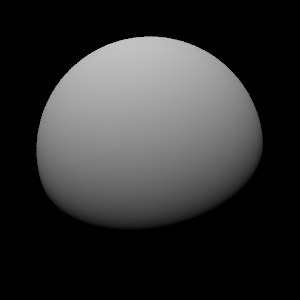You will need to rerun cmake whenever you add a file.

# Step 3: Implement Sphere Tracing

Sphere tracing was introduced to the graphics literature by John C. Hart in the paper we have linked in Step 1, but we will provide a layman description here.

You can implicitly describe a surface in 3-space as the set of points (x, y, z) such that f(x,y,z) = 0, for any continous 3D function f. If this function has the property that |f(x,y,z)| is always equal to the distance to the nearest zero point of the function, this function is known as a distance function.

A very simple example of a distance function is that of one for a sphere of radius R: f(x,y,z) = sqrt(x^2 + y^2 + z^2) - R. At every point outside of the sphere, f(x,y,z) gives the distance to the closest point on the sphere. At every point inside the sphere f(x,y,z) gives the negation of the distance to the closest point on the sphere. This type of distance function, which has "sides" of the surface, where the distances are negative, is known as a signed distance function. Any such function can be made "side-agnostic", by taking its absolute value.

Implement the distance evaluation function for a sphere in a function virtual Float DistanceEstimator::Evaluate(const Point3f& p) const. Have your DistanceEstimator constructor take in the sphere's radius as a parameter, but remove the other parameters left over from the sphere constructor.

Now for any distance function, we can intersect a ray with the implicit surface by:

• Evaluating the distance function at the beginning of the ray.
• Stepping along the ray by the value given by the distance function (increasing t by distance/length(ray.d)). It is important to remember that PBRT does not guarantee that the direction of its rays are normalized, so we need to compute t as the distance value divided by the length of the ray direction.
• By definition of the distance function, we haven't moved far enough to have crossed the surface (though we may be exactly at it)
• If we are within some small value (epsilon) of the surface we say we hit the surface
• If not, we take another step, the distance we step again given by the distance function
• Repeat until we get a hit or have gone past ray.tMax (we missed).

Note that this procedure will work not only for distance functions, but also any function that underestimates the distance to the nearest surface, and is non-zero whenever the true distance is non-zero. We call a function that gives the exact distance or less a distance estimator.

Below is a visual representation of the algorithm, taken from Figure 2 of "Enhanced Sphere Tracing" by Keinert et. al.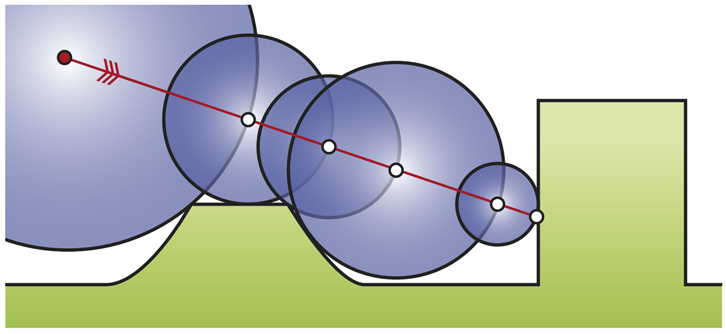We can implement this algorithm in DistanceEstimator::Intersect(). Now, if we have a hit, we have both the tHit value and the point of intersection. There is another output parameter to complete the Intersect() computation: SurfaceInteraction *isect.

This structure is fairly complicated, so let's take a look at the constructor below.

SurfaceInteraction(const Point3f& p, const Vector3f& pError,
const Point2f& uv, const Vector3f& wo,
const Vector3f& dpdu, const Vector3f& dpdv,
const Normal3f& dndu, const Normal3f& dndv, Float time,
const Shape *sh);


p is just the intersection point, we already have this value. sh is just a pointer to the shape (i.e. this). time can just be set to ray.time. wo is just the direction radiance scatters back along the ray, so you can set this to the negation of the ray direction. pError is the error bounds of the hit point, pbrt tries to guarantee that the "true" hitpoint, if we were calculating using the real numbers, would be in the box centered at p with extents equal to pError. You can set pError in an ad-hoc manner for now (interested students can also develop a principled approach for calculating pError based on the floating point error section of the textbook). One reasonable option for this assignment would be setting pError to 10 times the epsilon used for computing the hitpoints.

There is no natural "u-v" parameterization for a general implicit function, so the rest of the values are somewhat degenerate. We can just say uv is always the zero vector, and approximate our hit point locally by a plane, by saying the two derivatives of the normal dndu and dndv are zero.

Then all that is left is dpdu and dpdv. Since we have no "u-v" parameterization, these vectors have no real meaning. Unfortunately, we need the cross product (dpdu x dpdv) to evaluate to the normal of the surface, so that lighting calculations can have the surface normal. If we have a normal, its easy to get two vectors that fulfill this requirement, see the CoordinateSystem function in core/geometry.h.

Now we just have to be able to get the surface normal for a distance function. A nice property of signed distance functions is that the surface normal at a point is just the direction of the gradient at the point. We can approximate the gradient through finite differences. Feel free to come up with your own solution, or drop in the following function:

Vector3f DistanceEstimator::CalculateNormal(const Point3f& pos, float eps,
const Vector3f& defaultNormal) const {
const Vector3f v1 = Vector3f( 1.0,-1.0,-1.0);
const Vector3f v2 = Vector3f(-1.0,-1.0, 1.0);
const Vector3f v3 = Vector3f(-1.0, 1.0,-1.0);
const Vector3f v4 = Vector3f( 1.0, 1.0, 1.0);

const Vector3f normal = v1 * Evaluate( pos + v1*eps ) +
v2 * Evaluate( pos + v2*eps ) +
v3 * Evaluate( pos + v3*eps ) +
v4 * Evaluate( pos + v4*eps );
const Float length = normal.Length();

return length > 0 ? (normal/length) : defaultNormal;


}

We basically probe the distance function at small offsets from the function, to find the direction of maximum increase, which is the direction of the normal. The defaultNormal is used in case these evaluations perfectly cancel out and we get a 0-vector for the normal. This degenerate case will hopefully happen only rarely, one reasonable choice for defaultNormal is the negation of the ray direction.

In order to guarantee the intersection routine finishes in a bounded amount of time, we will want a maxIters parameter that bounds the number of iterations we step along the ray. This can in theory lead to erroneous misses; we can counteract this to an extent by making maxIters very large.

In total, there are four important parameters to a generic DistanceEstimator:

    int maxIters; // Number of steps along the ray until we give up (default 1000)
float hitEpsilon; // how close to the surface we must be before we say we "hit" it
float rayEpsilonMultiplier; // how much we multiply hitEpsilon by to get pError
float normalEpsilon; // The epsilon we send to CalculateNormal()


Make your DistanceEstimator class accept these parameters in CreateDistanceEstimatorShape(). It may be easier for you in later steps if you aggregate these 4 parameters into a struct.

When you finish Intersect(), make sure to correctly implement Area(), and ObjectBound(). You don't need a real implementation of Sample(), go ahead and make one that returns the default Iteraction() (like Cone's implementation). After all these changes, if everything goes well, you should be able to run scenes/assignment2/distanceestimatortest.pbrt again and get nearly identical results to that in Step 2.# Step 4: Refactor

Create a SphereDE subclass of DistanceEstimator; move DistanceEstimator's Evaluate(), Area(), and ObjectBound() there (make DistanceEstimator's Evaluate() a pure virtual function, and modify pbrt so that spherede.pbrt correctly produces an image of a sphere (you'll end up replacing CreateDistanceEstimatorShape() with CreateSphereDEShape() along the way).

By refactoring in this way, we have made it fairly simple to add different distance estimators to pbrt.

At this point, you should be able to run scenes/assignment2/spherede.pbrt and get the same result as in Step 3.# Step 5: Infinite Grid of Spheres

If we stopped at step 4, this would be a boring exercise. The strength of the distance estimator approach is in how it allows for extreme complexity from little data. Here we will implement an infinite grid of spheres, with only minor changes from SphereDE. First, create an InfiniteSphereGridDE, that is identical to SphereDE.

Now, we will modify Evaluate() to replicate the spheres everywhere. First, replace the radius parameter with 1.0 in the distance function (we will only have unit spheres for this project). Next replace the radius parameter that is stored in the object with a cellSize parameter. The idea is that we will be creating an infinite grid of cubic cells of side length cellSize, with a sphere centered in each one. Let the origin be at the center of a sphere. A point is always closest to the sphere in the cubic cell it is within, so the distance function only needs to compute the distance to the sphere in the cell the point is in! C++'s remainder() function might come in handy for this computation.

The area of an infinite number of spheres is of course infinite. Modify the Area() and ObjectBounds() functions to reflect the fact that we are tracing an infinite grid of spheres.

A small gotcha: pbrt does not seem to properly handle infinite bounding boxes. As a workaround, you can just make the bounding box really large. Of course, if you want to patch pbrt to properly handle bounding boxes instead, that would be great!

If you implemented everything correctly, you should get the following result when rendering scenes/assignment2/infinitegrid.pbrt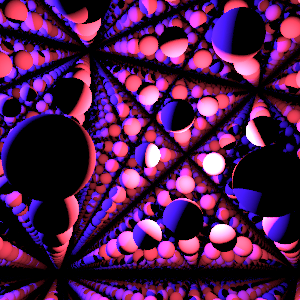Now, change the lighting/camera/parameters and render an interesting shot for your submission. Note that we are now tracing an infinite amount of geometry, something not possible with standard pbrt Shapes.

# Step 6: Mandelbulb

To demonstrate the versatility of the distance estimator approach, let's see how it works with a significantly more complicated distance function, one that has fine scale geometric detail down to the limits of floating point precision. A Mandelbulb is a fractal that has infinite surface area, and is completely contained in the unit sphere.

Simply add another subclass, MandelbulbDE. Have it take two integer parameters fractalIters (default 1000) and mandelbulbPower (default 8), and use the following Evaluate() function.

Float MandelbulbDE::Evaluate(const Point3f &p) const {
const float bailout = 2.0f;
const float Power = (float)mandelbulbPower;
Point3f z = p;
float dr = 1.0;
float r = 0.0;
for (int i = 0; i < fractalIters; i++) {
r = (z-Point3f(0,0,0)).Length();
if (r>bailout) break;

// convert to polar coordinates
float theta = acos(z.z/r);
float phi = atan2(z.y,z.x);
dr =  pow( r, Power-1.0)*Power*dr + 1.0;

// scale and rotate the point
float zr = pow( r,Power);
theta = theta*Power;
phi = phi*Power;

// convert back to cartesian coordinates
z = zr*Point3f(sin(theta)*cos(phi), sin(phi)*sin(theta), cos(theta));
z += p;
}
return 0.5*log(r)*r/dr;
}


If you are curious about how to derive distance estimators for iterative fractals (like the Mandelbulb one given above), you should read John C. Hart's Ray Tracing Deterministic Fractals. We are just using this example to demonstrate the power of distance estimators, so we will not dive into how to derive it here.

Modify pbrt to correctly read scenes/assignment2/mandelbulb.pbrt, and you should be able to render a result almost identical to this: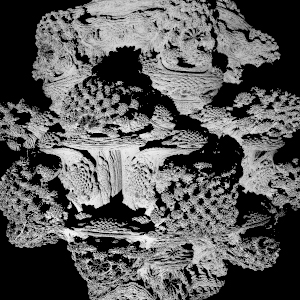Now, modify the lighting/camera/path length/parameters to find another interesting shot, and render that for your submission.

# Step 7: Another Distance Estimator

Create another distance estimator, anything that you want. Construct your own scene that uses your distance estimator, and render it. Try to make the result visually appealing.

If you are having trouble thinking of what to do for distance estimators, think about simple shapes. How might you define a plane distance estimator? a box? an infinite cylinder?

Maybe you could take a look at the link in the first image from this assignment for inspiration, and from this page you will find many distance estimators used for the first image.

# Step 8: Short Discussions

• Draw an example of a "bad" ray for sphere tracing (one that takes an inordinate amount of time to intersect), in the style of the sphere tracing image in Step 3. Feel free to scan in a hand-drawn image or use whatever software you like.
• You can find the union of multiple distance estimators at once by taking the min() of their distances at each point during the ray march. Describe what type of scene this could be more efficient than simply putting the individual distance estimators in a regular k-d tree. Then, describe what type scene this would be less efficient.
• What happens to your images if you set pError to zero in Intersect()? Why?

# Hints

• Seemingly small changes to the various "epsilon" parameters can lead to big differences in your result. Think carefully about what each epsilon parameter really means, geometrically.
• While developing, it may be useful to set IntersectP() to always return false, which has the effect of "turning off" shadows. That way you can separately debug the primary visibility intersection from shadow ray intersection.
• Compiling in debug mode and using a debugger (gdb/lldb/Visual Studio) can be helpful for tracking down errors.
• Test on small images and with less samples per pixel to save iteration time in your personal debug/modify/run loop.

# Submission

Once you are done with the assignment, convert your rendered images for steps 5 - 7 to png files for easier viewing using the following command:

imgtool convert --tonemap [imagename].exr [imagename].png


Make sure that the final png files are saved in the scenes/assignment2 directory of your repository.

Next, create a Submission.md file in the root of your repository that contains the following information:

• A description of the troubles you ran into during implementation, and how you overcame or worked around them.
• Embedded copies of your rendered images of the infinite grid of spheres, the mandelbulb, and your custom distance estimator.

When creating your Submission.md file, you can take advantage of GitHub's support for markdown syntax, which is detailed here. For example, given that your rendered images are stored in the scenes/assignment2 directory, you can include them in your Submission.md file as follows:

![InfiniteSpheres](scenes/assignment2/infinitegrid.png)


After creating Submission.md, push it to GitHub and ensure that your markdown is working correctly by viewing the file in your web browser.

In total, your submission should include the following files:

• All the code files you added and/or modified in pbrt (api.cpp, and the distance estimator class and subclasses).
• The new images you created in Steps 5-7.
• The new or modified scene files from Steps 5-7.
• Your Submission.md file.

Ensure that all your changes have been committed and pushed to GitHub:

git add [files] [you] [changed]

When you are ready to submit, create a pull request on GitHub from your assignment2 branch to your master branch, then add the TAs as reviewers of the pull request. DO NOT merge your pull request. Keep your master branch unchanged as a basis for future assignments.